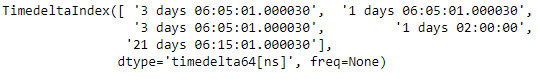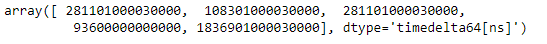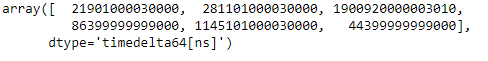# Python | Pandas TimedeltaIndex.get_values

Python is a great language for doing data analysis, primarily because of the fantastic ecosystem of data-centric python packages. Pandas is one of those packages and makes importing and analyzing data much easier.

Pandas` TimedeltaIndex.get_values()` function return the underlying data as an ndarray of the given TimedeltaIndex object.

Syntax : TimedeltaIndex.get_values()

Parameters : None

Return : ndarray

Example #1: Use `TimedeltaIndex.get_values()` function to return the underlying data of the given TimedeltaIndex object as an ndarray.

 `# importing pandas as pd ` `import` `pandas as pd ` ` `  `# Create the TimedeltaIndex object ` `tidx ``=` `pd.TimedeltaIndex(data ``=``[``'3 days 06:05:01.000030'``, ``'1 days 06:05:01.000030'``,  ` `                                ``'3 days 06:05:01.000030'``, ``'1 days 02:00:00'``, ` `                                                 ``'21 days 06:15:01.000030'``]) ` ` `  `# Print the TimedeltaIndex object ` `print``(tidx) `

Output :Now we will use the `TimedeltaIndex.get_values()` function to return the data of tidx as an ndarray

 `# return data as an ndarray ` `tidx.get_values() `

Output :As we can see in the output, the `TimedeltaIndex.get_values()` function has returned the data of the tidx object as an ndarray.

Example #2: Use `TimedeltaIndex.get_values()` function to return the underlying data of the given TimedeltaIndex object as an ndarray.

 `# importing pandas as pd ` `import` `pandas as pd ` ` `  `# Create the TimedeltaIndex object ` `tidx ``=` `pd.TimedeltaIndex(data ``=``[``'06:05:01.000030'``, ``'3 days 06:05:01.000030'``, ` `                                ``'22 day 2 min 3us 10ns'``, ``'+23:59:59.999999'``, ` `                             ``'13 days 06:05:01.000030'``, ``'+12:19:59.999999'``]) ` ` `  `# Print the TimedeltaIndex object ` `print``(tidx) `

Output :Now we will use the `TimedeltaIndex.get_values()` function to return the data of tidx as an ndarray

 `# return data as an ndarray ` `tidx.get_values() `

Output :As we can see in the output, the `TimedeltaIndex.get_values()` function has returned the data of the tidx object as an ndarray.

My Personal Notes arrow_drop_upCheck out this Author's contributed articles.

If you like GeeksforGeeks and would like to contribute, you can also write an article using contribute.geeksforgeeks.org or mail your article to contribute@geeksforgeeks.org. See your article appearing on the GeeksforGeeks main page and help other Geeks.

Please Improve this article if you find anything incorrect by clicking on the "Improve Article" button below.

Article Tags :

Be the First to upvote.

Please write to us at contribute@geeksforgeeks.org to report any issue with the above content.